Tapping into mathematics

Start this free course now. Just create an account and sign in. Enrol and complete the course for a free statement of participation or digital badge if available.

Free course

# 2.3 Some calculator conventions

Your calculator will interpret the order in which you press the keys, in a particular way. For example if you press the key sequence:

2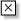3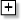4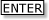(or if you have an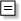key instead of, then press 234)

The calculator will first multiply 2 by 3 and then add 4 to give a result of 10. On the other hand if you enter the sequence:

234(or 234)

most scientific and graphics calculators give the answer 14, because they multiply 3 and 4 together before adding the result to 2.

The order in which a calculator handles calculations depends upon the operations involved. Multiplication and division are usually done before addition and subtraction.

Suppose you enter the sequence:

42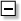79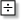3(or 42793)

Since the sequence contains multiplication, division, addition and subtraction, the calculator will follow a rule as to what to do first. The procedure will be built into the calculator (although some calculators allow you to change the rules). Most scientific and graphics calculators will perform multiplication and division before addition and subtraction. So the TI-84 performs 4 × 2 and 9 ÷ 3 first and then does the addition and subtraction. The calculation is set out below to illustrate this:

42793 is

8                −     7           +         3.

The calculator then performs the subtraction and addition in the order in which they appear (left to right) which gives 1 + 3 or 4.

Try the above calculation on your own calculator to see if it follows this process.

MU120_4MCAL